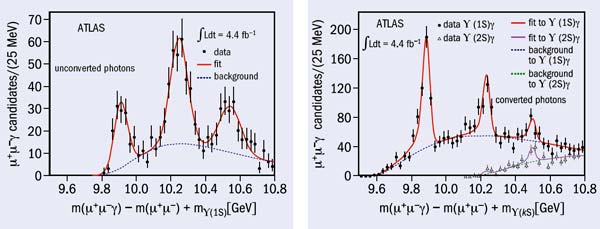# ATLAS discovers its first new particle

25 January 2012The spectrum of the χb states observed in three different ways with peaks, from the left, for the χb(1P), the χb(2P) and the new χb(3P). The plot on the left shows the spectrum for decays involving unconverted photons, while the right-hand plot shows spectra for decays involving converted photons. In the right-hand plot, the upper (red) curve shows the spectrum for χb decays to ϒ(1S), while the lower (brown) curve shows the spectrum for decays to ϒ(2S). (Only the χb(3P) peak appears distinctly in the lower spectrum because it is the only χb state with decays involving enough energy to be detected in this study.)

The ATLAS collaboration has announced the discovery of the χb(3P), which is a bound state of a bottom quark and bottom antiquark (bb).

Bound states of a heavy quark and its antiquark – collectively called quarkonium – are the QCD analogues of the hydrogen atom, with each particle corresponding to a different energy level. For bb states, the S states are the well known ϒ particles, while the P states are known as χb. As with the hydrogen atom, transitions between these states can be observed through the emission of a photon (γ).

The collaboration discovered the new state through the radiative transitions χb(3P) → ϒ(1S) + γ and χb(3P) → ϒ(2S) + γ, followed by the decay of the ϒ to two muons. The figure shows the spectrum of the χ states: the leftmost peak is the χb(1P), the middle one the χb(2P) and the rightmost the new state, χb(3P). The photons were detected either by the electromagnetic calorimeter (in which case they remained unconverted) or, if they had interacted with material and converted to an e+e pair, by the ATLAS tracking detectors.

Usually, a new particle is discovered in one or at most two channels, and the first observation is at the very edge of statistical significance. However, ATLAS has seen the χb(3P) with three different signatures, in both the ϒ(1S) and ϒ(2S) channels, and the peaks are unmistakable. The outstanding performance of both the LHC and the ATLAS detector made such a clear observation possible.

Also in analogy with atomic physics, the visible peaks contain internal structure from hyperfine splitting among states of different angular momentum. These could be resolved with future data samples.

Studying the energy levels of quarkonium states provides information about the forces that bind quarks together. One surprise is that the χb(3P) is slightly heavier than predicted, implying that the quark–antiquark pair is a little more loosely bound than expected. The χb(3P) is just at the very limit of being bound, so the quark and antiquark are about as far apart from each other as they can possibly be.